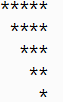Java Programs

Program 

Write a program to find the sum of 1st 10 odd natural numbers.

Program 

Write a program to find the sum of 1st 10 even natural numbers.

Program 

Write a program to find the sum of all 3-digit even natural numbers.

Program 

Write a program to find the sum of all 3 digit odd natural numbers, which are multiples of 5.

Program 

Write a program to input an integer and find its factorial. Factorial of a number is the product of all natural numbers till that number. For example factorial of 5 is 120 since 1×2×3×4×5=120.

Program 

Write a program to input an integer and print its factors.

Example

INPUT: Enter an integer:12

OUTPUT: Factors: 1 2 3 4 6 12

Program 

Write a program to input an integer and count the number of factors.

Program 

Write a program to input an integer and check whether it is a prime number or not.

Program 

Write a program to input 10 integers and find their sum.

Program 

Write a program to input 10 integers and find the sum of even numbers only.

Program 

Write a program to input 10 integers and find the sum of two digit as well as three digit numbers separately.

Program 

Write a program to input 10 integers and display the largest integer.

Program 

Write a program to input 10 integers and display the largest as well as the smallest integer.

Program 

Write a program to input 10 integers and display the largest even integer. In case there is no even integer, it should print “No even integer found”.

Program 

Write a program to input 10 integers and display the largest even and smallest odd integer.

Program 

Write a program to input 10 integers and check whether all the entered numbers are even numbers or not.

Program 

Write a program to input 10 integers and check whether all the entered numbers are same or not.

For Example,

INPUT: Enter 10 numbers: 10 12 13 234 45 34 67 78 76 12

OUTPUT: All numbers are not same.

INPUT: Enter 10 numbers: 12 12 12 12 12 12 12 12 12 12

OUTPUT: All numbers are same.

Program 

Write a program to input 10 integers and check whether the entered numbers are in ascending order or not.

For Example,

INPUT: Enter 10 numbers: 10 12 13 25 45 55 67 78 106 122

OUTPUT: The numbers are in ascending order.

INPUT: Enter 10 numbers: 25 34 56 67 12 32 43 21 23 111

OUTPUT: The numbers are not in ascending order.

Program 

Write a program to find the sum of 1st 10 numbers of Fibonacci series i.e. 1,1,2,3,5,8,13….

Fibonacci series is such a series which starting from 1 and 1, and subsequent numbers are the sum of the previous two numbers.

Program 

Write a program to print the first 15 numbers of the Pell series. Pell series is such a series which starts from 1 and 2 , and subsequent numbers is the sum of twice the previous number and the number previous to the previous number. Pell series: 1, 2, 5, 12, 29, 70, 169, 408, 985, 2378, 5741, 13860, …

Program 

Write a program to find the sum of 1st 10 numbers of Lucas series i.e. 2,1,3,4,7,11,18,….

Lucas series is such a series which starting from 2 and 1, and subsequent numbers are the sum of the previous two numbers.

Program 

Write a program to input an integer and check whether it is perfect, abundant or deficient number. If the sum of the factors excluding itself is equal to that number it is perfect, if greater than that number it is abundant and if less than that number it is deficient number.

Program 

Write a program to input two integers and check whether it forms an amicable pair or not. An amicable pair is such that, the sum of the factors excluding itself of one number is the other number and sum of the factors excluding itself of the other number is this number.

Example, (220, 284) . Since sum of factors excluding itself of:

220= 1+2+4+5+10+11+20+22+ 44+55+110=284

284= 1+ 2+4+71+142=220.

Program 

Write a program to pass an integer as argument and find the sum of its digits.

Program 

Write a program to pass an integer as argument and find the sum of odd digits and even digits separately.

Program 

Write a program to pass an integer as argument and find the average of its digits.

Program 

Write a program to pass an integer as argument and print the largest as well as smallest digit.

Program 

Write a program to input an integer and remove all the even digits from it.

For Example,

INPUT: Enter an integer: 1234

OUPUT: 13

Program 

Write a program to pass an integer as argument and check whether all digits in it are even digit or not.

Program 

Write a program to pass an integer as argument and check whether the digits are in ascending order or not.

Program 

Write a program to pass an integer as argument and check whether it is Armstrong number or not. Numbers whose sum of the cube of its digit is equal to the number itself is called Armstrong numbers. Example 153=13+53 +33.

Program 

Write a program to input an integer and check whether it is an automorphic, trimorphic or tri-automorphic number or not. A number n is said to be automorphic, if its square ends in n. For instance 5 is automorphic, because 52= 25, which ends in 5, 25 is automorphic, because 252=625, which ends in 25. A number n is called trimorphic if n3 ends in n. For example 493, = 117649, is trimorphic. A number n is called tri-automorphic if 3n2 ends in n; for example 667 is tri-automorphic because 3 × 6672, = 1334667, ends in 667.

Program 

Write a Program in Java to input a number and check whether it is a Harshad Number or Niven Number or not.

Harshad Number : In recreational mathematics, a Harshad number (or Niven number), is an integer (in base 10) that is divisible by the sum of its digits.

Let’s understand the concept of Harshad Number through the following example:

• The number 18 is a Harshad number in base 10, because the sum of the digits 1 and 8 is 9 (1 + 8 = 9), and 18 is divisible by 9 (since 18 % 9 = 0)
• The number 1729 is a Harshad number in base 10, because the sum of the digits 1 ,7, 2 and 9 is 19 (1 + 7 + 2 + 9 = 19), and 1729 is divisible by 19 (1729 = 19 * 91)
• The number 19 is not a Harshad number in base 10, because the sum of the digits 1 and 9 is 10 (1 + 9 = 10), and 19 is not divisible by 10 (since 19 % 10 = 9)

The first few Harshad numbers in base 10 are:

1, 2, 3, 4, 5, 6, 7, 8, 9, 10, 12, 18, 20, 21, 24, 27, 30, 36, 40, 42, 45, 48, 50, 54, 60, 63, 70, 72, 80, 81, 84, 90, 100, 102, 108, 110, 111, 112, 114, 117, 120, 126, 132, 133, 135, 140, 144, 150, 152, 153, 156, 162, 171, 180, 190, 192, 195, 198, 200 etc.

Sample:

Enter a number : 195

Enter a number : 194

194 is not a Harshad Number.

Enter a number : 190

Enter a number : 111

Program 

Write a program to input a number and check whether it is a Kaprekar number or not. Take a positive whole number n that has d number of digits. Take the square n and separate the result into two pieces: a right-hand piece that has d digits and a left-hand piece that has either d or d-1 digits. Add these two pieces together. If the result is n, then n is a Kaprekar number.

Examples are 9 (92 = 81, 8 + 1 = 9), 45 (452 = 2025, 20 + 25 = 45), and 297 (2972 = 88209, 88 + 209 = 297).

Program 

Write a program to input two integers and find their Least Common Multiple(L.C.M).

For Example,

INPUT: Enter 2 integers:

12

8

OUTPUT: L.C.M. = 24

Program 

Write a program to input two integers and find their Highest Common Factor(H.C.F).

For Example,

INPUT: Enter 2 integers:

12

8

OUTPUT: H.C.F. = 4

Program 

Write a menu driven class to accept a number from the user and check whether it is a Palindrome or a Perfect number.

a. Palindrome number- (a number is a Palindrome which when read in reverse order is same as read in the right order) Example: 11, 101, 151, etc.

b. Perfect number- (a number is called Perfect if it is equal to the sum of its factors other than the number itself.) Example: 6=1+2+3

Program 

Write a menu driven program to accept a number from the user and check whether it is ‘BUZZ’ number or to accept any two numbers and print the ‘GCD’ of them.

a. A BUZZ number is the number which either ends with 7 or divisible by 7.

b. GCD (Greatest Common Divisor) of two integers is calculated by continued division method. Divide the larger number by the smaller; the remainder then divides the previous divisor. The process is repeated till the remainder is zero. The divisor then results the GCD.

Program 

Write a menu driven program to accept a number and check and display whether it is a Prime Number or not OR an Automorphic Number or not.

a. Prime number : A number is said to be a prime number if it is divisible only by 1 and itself and not by any other number. Example : 3,5,7,11,13 etc.

b. Automorphic number : An automorphic number is the number which is contained in the last digit(s) of its square.

Example:

25 is an automorphic number as its square is 625 and 25 is present as the last two digits.

Program 

Write a program to input 10 integers and print the second largest number. Assume that there is at least one second largest number in the given set of integers.

For Example,

INPUT: Enter 10 integers:

12 35 46 22 34 56 78 89 23 21

OUTPUT: Second Largest Integer: 78

Program 

Write a program to find the sum of the following series:

S=1+(1+2)+(1+2+3)+(1+2+3+4)+(1+2+3+4+5)+…+(1+2+3+4+…+10)

(Please note that no nested loop is to be used)

Program 

Write a program to print the sum of negative numbers, sum of positive even numbers and sum of positive odd numbers from a list of numbers (N) entered by the user. The list terminates when the user enters a zero.

Program 

A game of dice is to be simulated for two players, each player gets a chance to throw his dice, and the value is added to his points, this process continues alternately until for the player whose added points equals to 20 and is declared the winner. Write a program to simulate this process using the random( ) function.

Program 

Write a program to input 10 integers and find the sum of the prime numbers only.

Program 

Write a program to input 10 integers and find the average of the prime numbers only.

Program 

Write a program to input 10 integers and find the sum of the perfect numbers only.

Program 

Write a program to input 10 integers and find the average of the perfect numbers only.

Program 

Write a program to input 10 integers and find the largest prime number if any.

Program 

Write a program to input 10 integers and find the smallest perfect number if any.

Program 

Write a program to generate all 2 digit prime numbers.

Program 

Write a program to generate all perfect numbers between 1 to 100.

Program 

Write a program to generate all 3 digit palindrome numbers.

Program 

WAP to Input an integer and check whether it is a special number or not.

A special number is such a number whose sum of the factorials of each digit is the number itself. For example, 145 is a special number because 1! + 4! + 5! = 1 + 24 +120 = 145. Special numbers are also called factorion.

Program 

Write a program to input a number and check whether it is a magic number or not. If you iterate the process of summing the squares of the decimal digits of the number, and if this process terminates in 1, then the original number is called a magic number. For example 55=> (5+5)=10=>(1 +0)=1.

Program 

Write a program to input an integer as argument, and print the number by having the digits arranged in ascending order.

Program 

Write a program to pass an integer as argument, and check whether all digits in it are unique or not.

Program 

Write a program to pass an integer as argument, and print the frequency of each digit in it.

Program 

Write a program to input 10 integers and find the sum of two-digit as well as three-digit numbers separately.

Program 

Write a program to input 10 numbers and print the largest prime number if any.

Program 

Write a program to generate all 2 digit twisted prime number. Twisted prime number is a number which is prime and its reverse is also prime. Example 13 and 31.

Program 

WAP to Input an integer and form a new number where the digits are in ascending order.

Program 

Write a program to pass an integer as argument and print the second largest digit

Program 

Write a program to input a number and check whether it is a happy number or not. If you iterate the process of summing the squares of the decimal digits of a number and if this process terminates in 1, then the original number is called a happy number. For example 7=> (72)=49=> (42+92)=97=>(92 +72)=130 =>(12 +32+02)=10 =>(12+02)= 1.

Program 

Write a program to input a number and print all its prime factors using prime factorization.

For Example,

INPUT: Enter an integer: 24

OUTPUT: Prime Factors using Prime Factorisation are:

2223

Program 

Write a program to input a number and check whether it is a Smith number or not. Smith number is such a number, the sum of whose digits equals the sum of the digits of its prime factors.

Smith number is a composite number in which the sum of its digits is equal to the sum of the digits of all its prime factors.

For Example 378 is a Smith Number as the sum of the digits of 378 are : 3+7+8 = 18. The prime factors of 378 are: 2, 3, 3, 3, 7 ( sum = 2+3+3+3+7 = 18).

Similarly 22 is a Smith Number as the sum of the digits are : 2+2=4. The prime factors of 22 are:

2 and 11 (Sum = 2+(1+1) = 4

Other Examples include 27, 58, 85, 94, 121, 166, 202, 265, etc.

Program 

Write a program to accept a number and check and display whether it is a spy number or not.

(A number is spy if the sum its digits equals the product of its digits.)

Example: consider the number 1124 , sum of the digits = 1 + 1 + 2 + 4 = 8

Product of the digits = 1 × 1 × 2 × 4 = 8

Program 

Write a program to input a number and check and print whether it is a Pronic number or not.

Pronic Number : A pronic number, oblong number, rectangular number or heteromecic number, is a number which is the product of two consecutive integers, that is, n (n + 1). The first few pronic numbers are:

0, 2, 6, 12, 20, 30, 42, 56, 72, 90, 110, 132, 156, 182, 210, 240, 272, 306, 342, 380, 420, 462 … etc.

Program 

Write a Program in Java to input a number and check whether it is a Disarium Number or not.

Note: A number will be called DISARIUM if sum of its digits powered with their respective position is equal to the original number.

For example 135 is a DISARIUM (Workings 11+32+53 = 135, some other DISARIUM are 89, 175, 518 etc)

Sample:

Enter a Number : 135

135 is a Disarium Number.

Enter a Number : 219

219 is not a Disarium Number.

Enter a Number : 89

89 is a Disarium Number.

Program 

Write a Program in Java to input a number and check whether it is an Automorphic Number or not.

Note: An automorphic number is a number which is present in the last digit(s) of its square.

Example: 25 is an automorphic number as its square is 625 and 25 is present as the last digits

Sample:

Enter a Number : 25

25 is an Automorphic Number.

Enter a Number : 6

6 is an Automorphic Number.

Enter a Number : 9

9 is not an Automorphic Number.

Program 

Write a Program in Java to input a number and check whether it is a Duck Number or not.

A Duck number is a number which has zeroes present in it, but there should be no zero present in the beginning of the number. For example 3210, 7056, 8430709 are all duck numbers whereas 08237, 04309 are not.

Program 

Write a Program in Java to input a number and check whether it is a Fascinating Number or not.

Fascinating Numbers: Some numbers of 3 digits or more exhibit a very interesting property. The property is such that, when the number is multiplied by 2 and 3, and both these products are concatenated with the original number, all digits from 1 to 9 are present exactly once, regardless of the number of zeroes.

Let’s understand the concept of Fascinating Number through the following example:

Consider the number 192, 192 x 1 = 192 192 x 2 = 384 192 x 3 = 576

Concatenating the results : 192384576

It could be observed that ‘192384576’ consists of all digits from 1 to 9 exactly once. Hence, it could be concluded that 192 is a Fascinating Number.

Some examples of fascinating Numbers are : 192, 219, 273, 327, 1902, 1920, 2019 etc.

Sample Data:

Enter a number : 273

273 is a Fascinating Number.

Enter a number : 853

853 is not a Fascinating Number.

Enter a number : 95

Number should be of atleast 3 digits.

Program 

Write a Program in Java to input a number and check whether it is an Evil Number or not.

Evil Number: An Evil number is a positive whole number which has even number of 1’s in its binary equivalent.

Example: Binary equivalent of 9 is 1001, which contains even number of 1’s. A few evil numbers are 3, 5, 6, 9….

Design a program to accept a positive whole number and find the binary equivalent of the number and count the number of 1’s in it and display whether it is a Evil number or not with an appropriate message. Output the result in format given below:

Example 1

INPUT : 15

BINARY EQUIVALENT : 1111

NO. OF 1’s : 4

OUTPUT : EVIL NUMBER

Example 2

INPUT : 26

BINARY EQUIVALENT : 11010

NO. OF 1’s : 3

OUTPUT : NOT AN EVIL NUMBER

Program 

Write a program in Java to print the sum of negative numbers, sum of positive odd numbers and sum of positive even numbers from a list of numbers entered by the user. The list terminates when the user enters a Zero.

Program 

A bank intends to design a program to display the denomination of an input amount, up to 5 digits. The available denomination with the bank are of rupees 1000 , 500 , 100 , 50 , 20 , 10 , 5 , 2 , and 1.

Design a program to accept the amount from the user and display the break-up in descending order of denomination. (i.e. preference should be given to the highest denomination available) along with the total number of notes. [Note: Only the denomination used should be displayed]. Also print the amount in words according to the digits.

Example 1

INPUT : 14856

OUTPUT        : ONE FOUR EIGHT FIVE SIX

DENOMINATION :

1000 x 14 = 14000

500 x 1 = 500

100 x 3 = 300

50 x 1 = 50

5 x 1 = 5

1 x 1 = 1

TOTAL = 14856

TOTAL NUMBER OF NOTES = 21

Example 2
INPUT : 6043
OUTPUT : SIX ZERO FOUR THREE
DENOMINATION :
1000 x 6 = 6000
20 x 2 = 40
2 x 1 = 2
1 x 1 = 1
TOTAL = 6043
TOTAL NUMBER OF NOTES = 10

Example 3

INPUT : 235001
OUTPUT : INVALID AMOUNT

Program 

Write a program to print Floyd's triangle as shown below:

1

2  3

4  5  6

7  8  9  10

11 12 13 14 15

Program 

Write a java program which accept the number of terms form user an print the the Fibonacci series up to given terms.

Program 

Write a java program to input any number and count the number of digits in number.

Program 

Write a java program to accept an integer number and print the frequency of each digit present in the number .

Sample:

Input : 3255435

Output:

Digit Frequency

2 1

3 2

4 1

5 3

Program 

Write a java program to accept an integer number to find out and print the difference between greatest and smallest digits presents in the number .

Program 

Write a Java program to print Following Pattern: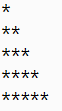Program 

Write a Java program to print Following Pattern: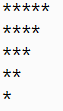Program 

Write a Java program to print Following Pattern: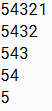Program 

Write a Java program to print Following Pattern: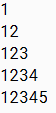Program 

Write a Java program to print Following Pattern: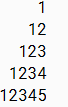Program 

Write a Java program to print Following Pattern: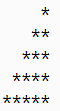Program 

Write a Java program to print Following Pattern: